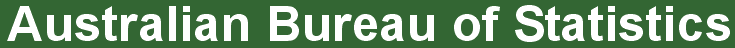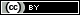ABS Home
 Statistical Language - Measures of ShapeMeasures of Shape

What is a measure of shape?

Measures of shape describe the distribution (or pattern) of the data within a dataset.

The distribution shape of quantitative data can be described as there is a logical order to the values, and the 'low' and 'high' end values on the x-axis of the histogram are able to be identified.

The distribution shape of a qualitative data cannot be described as the data are not numeric.

What are the shapes of a dataset?

A distribution of data item values may be symmetrical or asymmetrical. Two common examples of symmetry and asymmetry are the 'normal distribution' and the 'skewed distribution'.

In a symmetrical distribution the two sides of the distribution are a mirror image of each other.

A normal distribution is a true symmetric distribution of observed values.

When a histogram is constructed on values that are normally distributed, the shape of columns form a symmetrical bell shape. This is why this distribution is also known as a 'normal curve' or 'bell curve'.

The following graph is an example of a normal distribution:If represented as a 'normal curve' (or bell curve) the graph would take the following shape (where µ = mean, and σ = standard deviation):Key features of the normal distribution:
• symmetrical shape
• mode, median and mean are the same and are together in the centre of the curve
• there can only be one mode (i.e. there is only one value which is most frequently observed)
• most of the data are clustered around the centre, while the more extreme values on either side of the centre become less rare as the distance from the centre increases (i.e. About 68% of values lie within one standard deviation (σ) away from the mean; about 95% of the values lie within two standard deviations; and about 99.7% are within three standard deviations. This is known as the empirical rule or the 3-sigma rule.)

In an asymmetrical distribution the two sides will not be mirror images of each other.

Skewness is the tendency for the values to be more frequent around the high or low ends of the x-axis.

When a histogram is constructed for skewed data it is possible to identify skewness by looking at the shape of the distribution.

For example:

A distribution is said to be positively skewed when the tail on the right side of the histogram is longer than the left side. Most of the values tend to cluster toward the left side of the x-axis (i.e. the smaller values) with increasingly fewer values at the right side of the x-axis (i.e. the larger values).A distribution is said to be negatively skewed when the tail on the left side of the histogram is longer than the right side. Most of the values tend to cluster toward the right side of the x-axis (i.e. the larger values), with increasingly less values on the left side of the x-axis (i.e. the smaller values).Key features of the skewed distribution:
• asymmetrical shape
• mean and median have different values and do not all lie at the centre of the curve
• there can be more than one mode
• the distribution of the data tends towards the high or low end of the dataset

What are the other possible distribution shapes?

Other distributions include uni-modal, bi-modal, or multimodal.

A uni-modal distribution occurs if there is only one 'peak' (a highest point) in the distribution, as seen in the previous histograms. This means there is one mode (a value that occurs more frequently than any other) for the data item (variable).

The distribution shape of the data in the histogram below is bi-modal because there are two modes (two values that occur more frequently than any other) for the data item (variable).Why are measures of shape useful?

The shape of the distribution can assist with identifying other descriptive statistics, such as which measure of central tendency is appropriate to use.

If the data are normally distributed, the mean, median and mode are all equal, and therefore are all appropriate measure of centre central tendency.

If data are skewed, the median may be a more appropriate measure of central tendency.

Further information:

 Privacy | Disclaimer | Feedback || © Copyright| Sitemap| Online Security